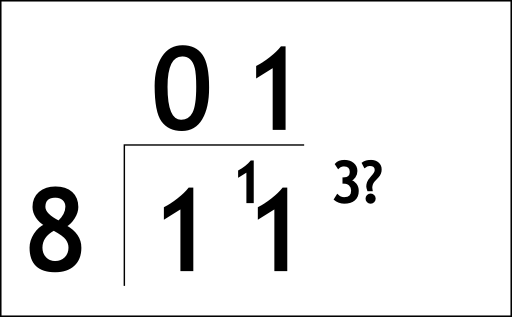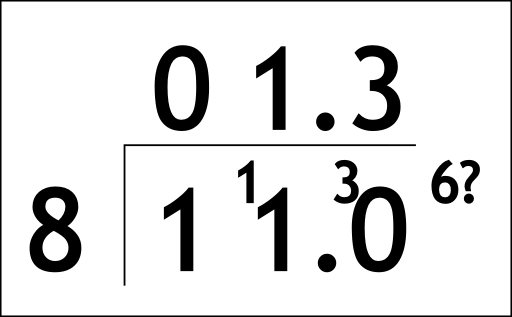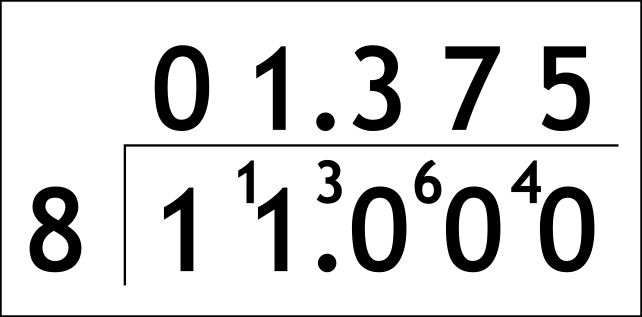The Singing Hedgehog Guide to:
Decimal Division 2

Now let's look at what happens when a sum does not divide exactly.

We will do 11÷8

Now we start as normal by the bus stop methodand we have three left over. We could now write this as three eighths.

Instead we turn our 11 into 11.0 which is of course the same value.
We put a matching decimal point in the answer too.

We now have somewhere to put our 3:We have the same problem with the 6. All we do is keep bunging on zeroes!next page

decimal index